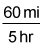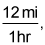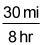##### SAT: 1,001 Practice Questions For DummiesIf you are confronted by an SAT Math exam question involving time, rate, and distance, there's a handy little formula you can use to solve it: distance equals rate multiplied by time, or D = RT.

The following practice questions are a little more challenging because they ask you to find average overall speed; fortunately, it's still all about applying the D=RT formula!

## Practice questions

1. Henry skates uphill on a paved path at an average speed of 10 miles per hour and downhill on the same path at an average speed of 15 miles per hour. What is his average (arithmetic mean) skating speed for the entire route, in miles per hour?
2. Yan swims upstream at an average speed of 3 knots (nautical miles per hour) and downstream on the same route at an average speed of 5 knots. What is her average (arithmetic mean) swimming speed for the entire route, in knots?

1. The correct answer is 12 or 60/5. To find the average speed of a trip, place the total distance over the total time. Pick a number for the distance. To simplify the math, use the lowest common multiple of the two speeds, 10 and 15, which is 30. If Henry skated 30 miles uphill at 10 miles per hour, he skated for 3 hours. If he skated 30 miles back at 15 miles per hour, he skated for 2 hours. Now you have the total distance and total time, which is 60 miles over 5 hours. Set it up as a fraction:You can reduce the fraction toor 12 mph.
2. The correct answer is 3.75 or 30/8 or 15/4. To find the average speed of a trip, place the total distance over the total time. Pick a number for the distance. To simplify the math, use the lowest common multiple of the two speeds, 3 and 5, which is 15. If Yan swam uphill, a distance of 15 nautical miles at 3 knots, she swam for 5 hours. If she swam back at 5 knots, she swam for 3 hours. Now you have the total distance and total time, which is 30 nautical miles over 8 hours. Set it up as a fraction:This equals 15/4 or 3.75 knots.